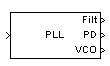# Phase-Locked Loop

(To be removed) Implement phase-locked loop to recover phase of input signal

Phase-Locked Loop will be removed in a future release. To design voltage-controlled oscillators (VCOs) and phase-locked loops (PLLs), use the Phase-Locked Loops (Mixed-Signal Blockset) blocks.

## Library

Components sublibrary of Synchronization

•## Description

The Phase-Locked Loop (PLL) block is a feedback control system that automatically adjusts the phase of a locally generated signal to match the phase of an input signal. This block is most appropriate when the input is a narrowband signal.

This PLL has these three components:

• A multiplier used as a phase detector.

• A filter. You specify the filter transfer function using the Lowpass filter numerator and Lowpass filter denominator parameters. Each is a vector that gives the respective polynomial's coefficients in order of descending powers of s.

To design a filter, you can use functions such as `butter`, `cheby1`, and `cheby2` in Signal Processing Toolbox™ software. The default filter is a Chebyshev type II filter whose transfer function arises from the command below.

`[num, den] = cheby2(3,40,100,'s')`

• A voltage-controlled oscillator (VCO). You specify characteristics of the VCO using the VCO quiescent frequency, VCO initial phase, and VCO output amplitude parameters.

This block accepts a sample-based scalar input signal. The input signal represents the received signal. The three output ports produce:

• The output of the filter

• The output of the phase detector

• The output of the VCO

## Parameters

Lowpass filter numerator

The numerator of the lowpass filter transfer function, represented as a vector that lists the coefficients in order of descending powers of s.

Lowpass filter denominator

The denominator of the lowpass filter transfer function, represented as a vector that lists the coefficients in order of descending powers of s.

VCO input sensitivity (Hz/V)

This value scales the input to the VCO and, consequently, the shift from the VCO quiescent frequency value. The units of VCO input sensitivity are Hertz per volt.

VCO quiescent frequency (Hz)

The frequency of the VCO signal when the voltage applied to it is zero. This should match the carrier frequency of the input signal.

The initial phase of the VCO signal.

VCO output amplitude

The amplitude of the VCO signal.